# Home Work Problems and Solutions: 1-6

 Home Work Problems and Solutions: 1-6 At the instant of Fig. 11-42, a 2.0 kg particle P has a position vector of magnitude 3.0 m and angle θ1 = 45° and a velocity vector of magnitude 4.0 m/s and angle θ2 30°. Force of magnitude 2.0 N and angle θ3 =30°, acts on P. All three vectors lie in the xy plane. About the origin, what are the (a) magnitude and (b) direction of the angular momentum of P and the (c) magnitude and (d) direction of the torque acting on P? (HR 11-28)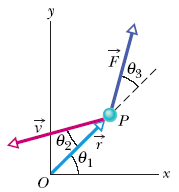Sol: We note that the component of perpendicular to has magnitude v sin  where = 30°. A similar observation applies to . (a) Eq. 11-20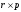leads to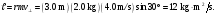(b) Using the right-hand rule for vector products, we findpoints out of the page, or along the +z axis, perpendicular to the plane of the figure. (c) Eq. 10-38 leads to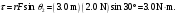(d) Using the right-hand rule for vector products, we find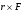is also out of the page, or along the +z axis, perpendicular to the plane of the figure. A track is mounted on a large wheel that is free to turn with negligible friction about a vertical axis (Fig. 11-49). A toy train of mass m is placed on the track and, with the system initially at rest, the train’s electrical power is turned on.The train reaches speed 0.15 m/s with respect to the track. What is the angular speed of the wheel if its mass is 1.1m and its radius is 0.43 m? (Treat the wheel as a hoop, and neglect the mass of the spokes and hub.) (HR 11-49) Sol: No external torques act on the system consisting of the train and wheel, so the total angular momentum of the system (which is initially zero) remains zero. Let I = MR2 be the rotational inertia of the wheel. Its final angular momentum is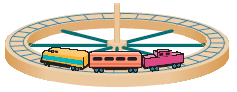Lf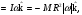where is up in Fig. 11-47 and that last step (with the minus sign) is done in recognition that the wheel’s clockwise rotation implies a negative value for . The linear speed of a point on the track is R and the speed of the train (going counterclockwise in Fig. 11-47 with speed relative to an outside observer) is therefore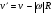where v is its speed relative to the tracks. Consequently, the angular momentum of the train is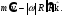Conservation of angular momentum yields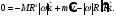When this equation is solved for the angular speed, the result is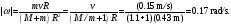Download 463.82 Kb.Share with your friends: